# Capital structure.pptx

29. May 2023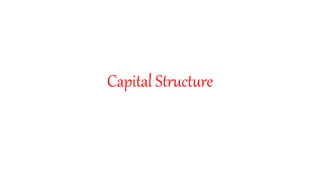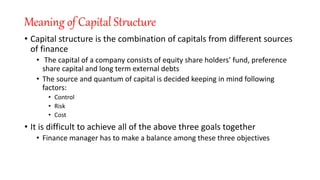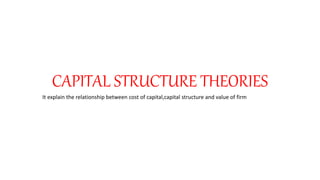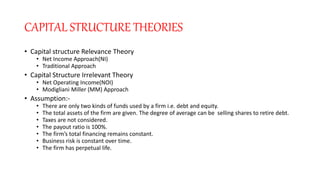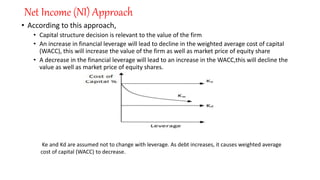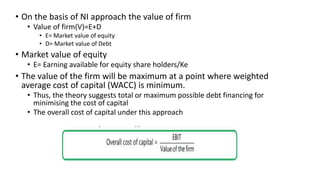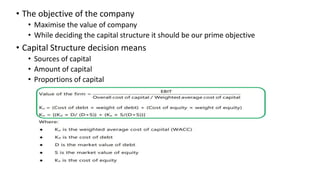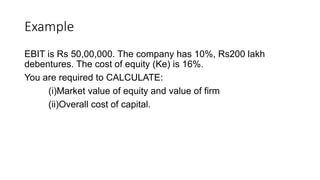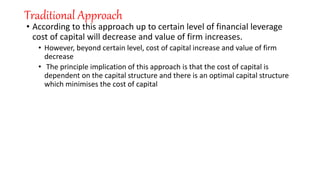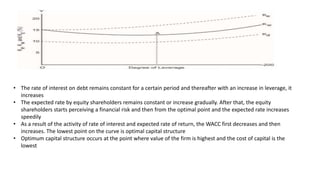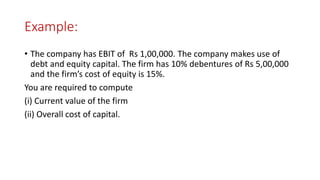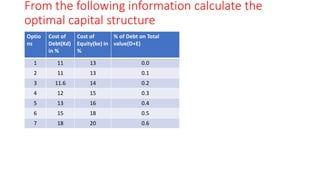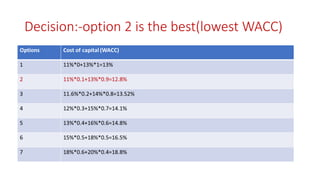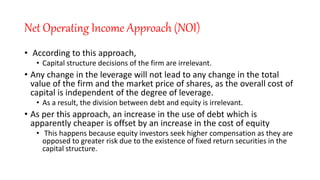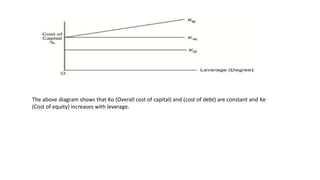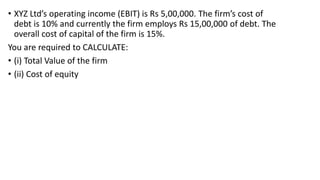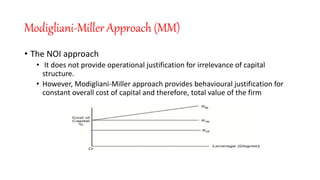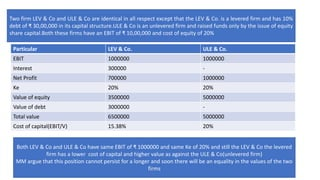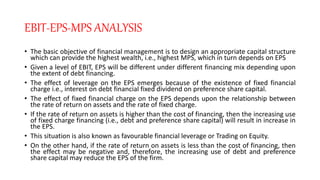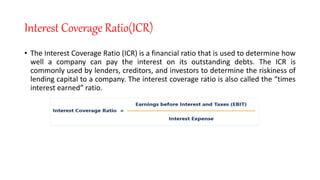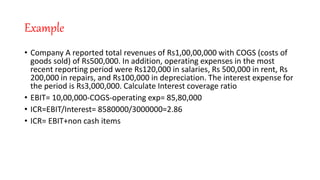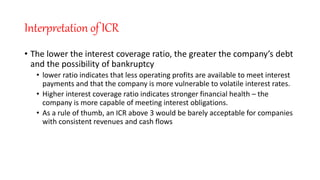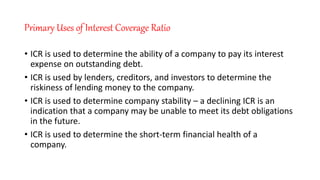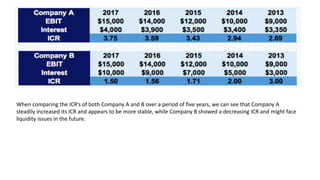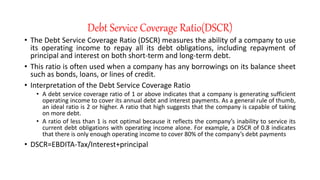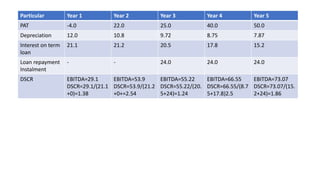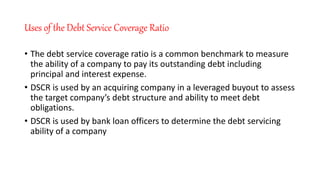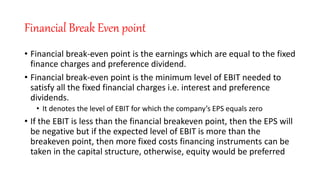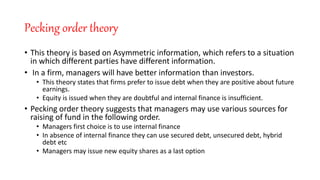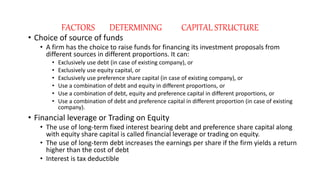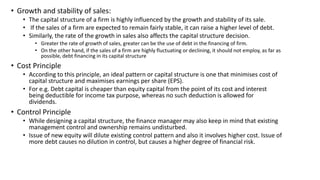1 von 31

### Capital structure.pptx

• 1. Capital Structure
• 2. Meaning of Capital Structure • Capital structure is the combination of capitals from different sources of finance • The capital of a company consists of equity share holders’ fund, preference share capital and long term external debts • The source and quantum of capital is decided keeping in mind following factors: • Control • Risk • Cost • It is difficult to achieve all of the above three goals together • Finance manager has to make a balance among these three objectives
• 3. CAPITAL STRUCTURE THEORIES It explain the relationship between cost of capital,capital structure and value of firm
• 4. CAPITAL STRUCTURE THEORIES • Capital structure Relevance Theory • Net Income Approach(NI) • Traditional Approach • Capital Structure Irrelevant Theory • Net Operating Income(NOI) • Modigliani Miller (MM) Approach • Assumption:- • There are only two kinds of funds used by a firm i.e. debt and equity. • The total assets of the firm are given. The degree of average can be selling shares to retire debt. • Taxes are not considered. • The payout ratio is 100%. • The firm’s total financing remains constant. • Business risk is constant over time. • The firm has perpetual life.
• 5. Net Income (NI) Approach • According to this approach, • Capital structure decision is relevant to the value of the firm • An increase in financial leverage will lead to decline in the weighted average cost of capital (WACC), this will increase the value of the firm as well as market price of equity share • A decrease in the financial leverage will lead to an increase in the WACC,this will decline the value as well as market price of equity shares. Ke and Kd are assumed not to change with leverage. As debt increases, it causes weighted average cost of capital (WACC) to decrease.
• 6. • On the basis of NI approach the value of firm • Value of firm(V)=E+D • E= Market value of equity • D= Market value of Debt • Market value of equity • E= Earning available for equity share holders/Ke • The value of the firm will be maximum at a point where weighted average cost of capital (WACC) is minimum. • Thus, the theory suggests total or maximum possible debt financing for minimising the cost of capital • The overall cost of capital under this approach
• 7. • The objective of the company • Maximise the value of company • While deciding the capital structure it should be our prime objective • Capital Structure decision means • Sources of capital • Amount of capital • Proportions of capital
• 8. Example EBIT is Rs 50,00,000. The company has 10%, Rs200 lakh debentures. The cost of equity (Ke) is 16%. You are required to CALCULATE: (i)Market value of equity and value of firm (ii)Overall cost of capital.
• 9. Traditional Approach • According to this approach up to certain level of financial leverage cost of capital will decrease and value of firm increases. • However, beyond certain level, cost of capital increase and value of firm decrease • The principle implication of this approach is that the cost of capital is dependent on the capital structure and there is an optimal capital structure which minimises the cost of capital
• 10. • The rate of interest on debt remains constant for a certain period and thereafter with an increase in leverage, it increases • The expected rate by equity shareholders remains constant or increase gradually. After that, the equity shareholders starts perceiving a financial risk and then from the optimal point and the expected rate increases speedily • As a result of the activity of rate of interest and expected rate of return, the WACC first decreases and then increases. The lowest point on the curve is optimal capital structure • Optimum capital structure occurs at the point where value of the firm is highest and the cost of capital is the lowest
• 11. Example: • The company has EBIT of Rs 1,00,000. The company makes use of debt and equity capital. The firm has 10% debentures of Rs 5,00,000 and the firm’s cost of equity is 15%. You are required to compute (i) Current value of the firm (ii) Overall cost of capital.
• 12. From the following information calculate the optimal capital structure Optio ns Cost of Debt(Kd) in % Cost of Equity(ke) in % % of Debt on Total value(D+E) 1 11 13 0.0 2 11 13 0.1 3 11.6 14 0.2 4 12 15 0.3 5 13 16 0.4 6 15 18 0.5 7 18 20 0.6
• 13. Decision:-option 2 is the best(lowest WACC)
• 14. Net Operating Income Approach (NOI) • According to this approach, • Capital structure decisions of the firm are irrelevant. • Any change in the leverage will not lead to any change in the total value of the firm and the market price of shares, as the overall cost of capital is independent of the degree of leverage. • As a result, the division between debt and equity is irrelevant. • As per this approach, an increase in the use of debt which is apparently cheaper is offset by an increase in the cost of equity • This happens because equity investors seek higher compensation as they are opposed to greater risk due to the existence of fixed return securities in the capital structure.
• 15. The above diagram shows that Ko (Overall cost of capital) and (cost of debt) are constant and Ke (Cost of equity) increases with leverage.
• 16. • XYZ Ltd’s operating income (EBIT) is Rs 5,00,000. The firm’s cost of debt is 10% and currently the firm employs Rs 15,00,000 of debt. The overall cost of capital of the firm is 15%. You are required to CALCULATE: • (i) Total Value of the firm • (ii) Cost of equity
• 17. Modigliani-Miller Approach (MM) • The NOI approach • It does not provide operational justification for irrelevance of capital structure. • However, Modigliani-Miller approach provides behavioural justification for constant overall cost of capital and therefore, total value of the firm
• 18. Particular LEV & Co. ULE & Co. EBIT 1000000 1000000 Interest 300000 - Net Profit 700000 1000000 Ke 20% 20% Value of equity 3500000 5000000 Value of debt 3000000 - Total value 6500000 5000000 Cost of capital(EBIT/V) 15.38% 20% Two firm LEV & Co and ULE & Co are identical in all respect except that the LEV & Co. is a levered firm and has 10% debt of ₹ 30,00,000 in its capital structure.ULE & Co is an unlevered firm and raised funds only by the issue of equity share capital.Both these firms have an EBIT of ₹ 10,00,000 and cost of equity of 20% Both LEV & Co and ULE & Co have same EBIT of ₹ 1000000 and same Ke of 20% and still the LEV & Co the levered firm has a lower cost of capital and higher value as against the ULE & Co(unlevered firm) MM argue that this position cannot persist for a longer and soon there will be an equality in the values of the two firms
• 19. EBIT-EPS-MPS ANALYSIS • The basic objective of financial management is to design an appropriate capital structure which can provide the highest wealth, i.e., highest MPS, which in turn depends on EPS • Given a level of EBIT, EPS will be different under different financing mix depending upon the extent of debt financing. • The effect of leverage on the EPS emerges because of the existence of fixed financial charge i.e., interest on debt financial fixed dividend on preference share capital. • The effect of fixed financial charge on the EPS depends upon the relationship between the rate of return on assets and the rate of fixed charge. • If the rate of return on assets is higher than the cost of financing, then the increasing use of fixed charge financing (i.e., debt and preference share capital) will result in increase in the EPS. • This situation is also known as favourable financial leverage or Trading on Equity. • On the other hand, if the rate of return on assets is less than the cost of financing, then the effect may be negative and, therefore, the increasing use of debt and preference share capital may reduce the EPS of the firm.
• 20. Interest Coverage Ratio(ICR) • The Interest Coverage Ratio (ICR) is a financial ratio that is used to determine how well a company can pay the interest on its outstanding debts. The ICR is commonly used by lenders, creditors, and investors to determine the riskiness of lending capital to a company. The interest coverage ratio is also called the “times interest earned” ratio.
• 21. Example • Company A reported total revenues of Rs1,00,00,000 with COGS (costs of goods sold) of Rs500,000. In addition, operating expenses in the most recent reporting period were Rs120,000 in salaries, Rs 500,000 in rent, Rs 200,000 in repairs, and Rs100,000 in depreciation. The interest expense for the period is Rs3,000,000. Calculate Interest coverage ratio • EBIT= 10,00,000-COGS-operating exp= 85,80,000 • ICR=EBIT/Interest= 8580000/3000000=2.86 • ICR= EBIT+non cash items • EBIT:-10,000,000-500000-120000-500000-200000-100000=8580,000 • Interest:-30,00,000 • ICR=8580000/3000000=2.86
• 22. Interpretation of ICR • The lower the interest coverage ratio, the greater the company’s debt and the possibility of bankruptcy • lower ratio indicates that less operating profits are available to meet interest payments and that the company is more vulnerable to volatile interest rates. • Higher interest coverage ratio indicates stronger financial health – the company is more capable of meeting interest obligations. • As a rule of thumb, an ICR above 3 would be barely acceptable for companies with consistent revenues and cash flows
• 23. Primary Uses of Interest Coverage Ratio • ICR is used to determine the ability of a company to pay its interest expense on outstanding debt. • ICR is used by lenders, creditors, and investors to determine the riskiness of lending money to the company. • ICR is used to determine company stability – a declining ICR is an indication that a company may be unable to meet its debt obligations in the future. • ICR is used to determine the short-term financial health of a company.
• 24. When comparing the ICR’s of both Company A and B over a period of five years, we can see that Company A steadily increased its ICR and appears to be more stable, while Company B showed a decreasing ICR and might face liquidity issues in the future.
• 25. Debt Service Coverage Ratio(DSCR) • The Debt Service Coverage Ratio (DSCR) measures the ability of a company to use its operating income to repay all its debt obligations, including repayment of principal and interest on both short-term and long-term debt. • This ratio is often used when a company has any borrowings on its balance sheet such as bonds, loans, or lines of credit. • Interpretation of the Debt Service Coverage Ratio • A debt service coverage ratio of 1 or above indicates that a company is generating sufficient operating income to cover its annual debt and interest payments. As a general rule of thumb, an ideal ratio is 2 or higher. A ratio that high suggests that the company is capable of taking on more debt. • A ratio of less than 1 is not optimal because it reflects the company’s inability to service its current debt obligations with operating income alone. For example, a DSCR of 0.8 indicates that there is only enough operating income to cover 80% of the company’s debt payments • DSCR=EBDITA-Tax/Interest+principal
• 26. Particular Year 1 Year 2 Year 3 Year 4 Year 5 PAT -4.0 22.0 25.0 40.0 50.0 Depreciation 12.0 10.8 9.72 8.75 7.87 Interest on term loan 21.1 21.2 20.5 17.8 15.2 Loan repayment Instalment - - 24.0 24.0 24.0 DSCR EBITDA=29.1 DSCR=29.1/(21.1 +0)=1.38 EBITDA=53.9 DSCR=53.9/(21.2 +0+=2.54 EBITDA=55.22 DSCR=55.22/(20. 5+24)=1.24 EBITDA=66.55 DSCR=66.55/(8.7 5+17.8)2.5 EBITDA=73.07 DSCR=73.07/(15. 2+24)=1.86
• 27. Uses of the Debt Service Coverage Ratio • The debt service coverage ratio is a common benchmark to measure the ability of a company to pay its outstanding debt including principal and interest expense. • DSCR is used by an acquiring company in a leveraged buyout to assess the target company’s debt structure and ability to meet debt obligations. • DSCR is used by bank loan officers to determine the debt servicing ability of a company
• 28. Financial Break Even point • Financial break-even point is the earnings which are equal to the fixed finance charges and preference dividend. • Financial break-even point is the minimum level of EBIT needed to satisfy all the fixed financial charges i.e. interest and preference dividends. • It denotes the level of EBIT for which the company’s EPS equals zero • If the EBIT is less than the financial breakeven point, then the EPS will be negative but if the expected level of EBIT is more than the breakeven point, then more fixed costs financing instruments can be taken in the capital structure, otherwise, equity would be preferred
• 29. Pecking order theory • This theory is based on Asymmetric information, which refers to a situation in which different parties have different information. • In a firm, managers will have better information than investors. • This theory states that firms prefer to issue debt when they are positive about future earnings. • Equity is issued when they are doubtful and internal finance is insufficient. • Pecking order theory suggests that managers may use various sources for raising of fund in the following order. • Managers first choice is to use internal finance • In absence of internal finance they can use secured debt, unsecured debt, hybrid debt etc • Managers may issue new equity shares as a last option
• 30. FACTORS DETERMINING CAPITAL STRUCTURE • Choice of source of funds • A firm has the choice to raise funds for financing its investment proposals from different sources in different proportions. It can: • Exclusively use debt (in case of existing company), or • Exclusively use equity capital, or • Exclusively use preference share capital (in case of existing company), or • Use a combination of debt and equity in different proportions, or • Use a combination of debt, equity and preference capital in different proportions, or • Use a combination of debt and preference capital in different proportion (in case of existing company). • Financial leverage or Trading on Equity • The use of long-term fixed interest bearing debt and preference share capital along with equity share capital is called financial leverage or trading on equity. • The use of long-term debt increases the earnings per share if the firm yields a return higher than the cost of debt • Interest is tax deductible
• 31. • Growth and stability of sales: • The capital structure of a firm is highly influenced by the growth and stability of its sale. • If the sales of a firm are expected to remain fairly stable, it can raise a higher level of debt. • Similarly, the rate of the growth in sales also affects the capital structure decision. • Greater the rate of growth of sales, greater can be the use of debt in the financing of firm. • On the other hand, if the sales of a firm are highly fluctuating or declining, it should not employ, as far as possible, debt financing in its capital structure • Cost Principle • According to this principle, an ideal pattern or capital structure is one that minimises cost of capital structure and maximises earnings per share (EPS). • For e.g. Debt capital is cheaper than equity capital from the point of its cost and interest being deductible for income tax purpose, whereas no such deduction is allowed for dividends. • Control Principle • While designing a capital structure, the finance manager may also keep in mind that existing management control and ownership remains undisturbed. • Issue of new equity will dilute existing control pattern and also it involves higher cost. Issue of more debt causes no dilution in control, but causes a higher degree of financial risk.

### Hinweis der Redaktion

1. EBITDA:-29.1 29.1/(21.1)=1.38 EBITDA:-54 54/21.2=2.55 EBITDA:-55.22 55.22/(20.5+24)=1.24 EBITDA:-66.55 66.55/(17.8+24) =1.59 EBITDA:-73.07 73.07/(15.2+24) 1.86